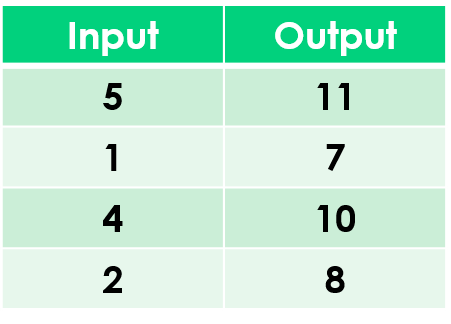1
visibility

Find the rule from the table below:• A

Add 6

• B

Add 3

The correct answer is "add 6".

Please Note:
Addition is the operation that increases a number by adding to it.

Solution:

To find the correct rule, we consider the given table.

We observe that the given rule makes each number increase. This implies addition.

We try adding 6 to each input number:

5 + 6 = 11
1 + 6 = 7
4 + 6 = 10
2 + 6 = 8

On adding 6 to the input numbers, we get the corresponding output numbers.

So, the rule is "add 6”.